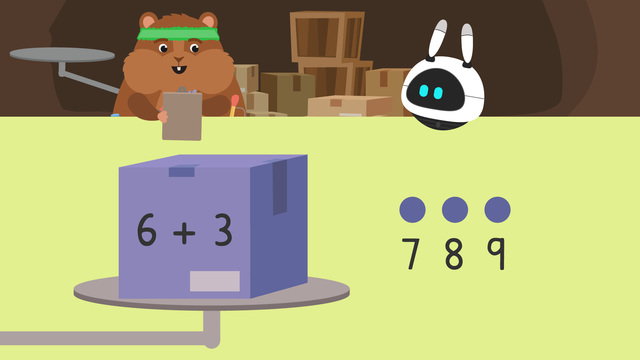# Equal / Not equalRating

Ø 5.0 / 1 ratings

The authorsTeam Digital
Equal / Not equal
CCSS.MATH.CONTENT.1.OA.D.7

## Basics on the topicEqual / Not equal

### What is Equal, Not equal?

In this video Mr. Squeaks has just gotten his latest shipment of supplies! He’s excited to start inventing all sorts of amazing things, but first he needs to organize all of these boxes. He decides to organize them by weight to see which ones are equal. Read more below to learn about not less or equal.

#### Equal or Not Equal?

How can you know if two expressions are equal or not equal? We can use expressions and counting to help! When trying to figure out if two or more expressions are equal, start by solving the first expression.

In this video, we add two addends together to find the sum. Next, solve the second expression. You can do this using a variety of different strategies, remember that in this video we solved the expressions by counting up. Finally, compare the answers, or sums, to see if they are equal or not. If the sums are the same number, the expressions are equal! If not, they are not equal.

#### Equal Not Equal Practice

Let’s practice finding if expressions are equal or not equal with the example below.

Take a look at the expressions on these boxes. Do you think that they are equal or not equal? Remember to solve each expression and compare the answers to see if they are equal or not equal.

These expressions are equal!

Let’s try one more.

Do you think that the expressions above are equal or not equal? Don’t forget to solve the expressions and compare the answers!

6 + 2 does not equal 7 + 3. The sum of 6 + 2 is 8 and is not equal to the sum of 7 + 3, which is 10. These expressions are not equal! How do you write the not equal symbol? The not equal symbol looks like =/=. =/= does not equal means the sums are different.

#### Equal and Not Equal - Summary

When trying to figure out if expressions are equal to or not equal to…

• Step one: Solve the first expression

• Step two: Solve the second expression

• Step three: Compare the sums! If they are the same number, it is equal!

#### Equal and Not Equal – Additional Practice

At the end of the video, there are exercises for continued practice of making change through equal or not equal worksheets.